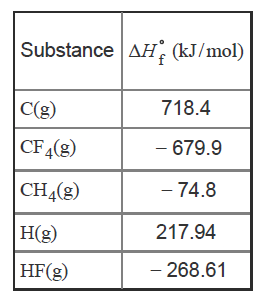# Problem: Calculate the enthalpy change, ΔH°, for the reverse of the formation of methane:CH4(g) → C(s) + 2 H2(g)Express your answer to one decimal place and include the appropriate units.Use the data below to answer the questions.Keep in mind that the enthalpy of formation of an element in its standard state is zero.Imagine a hypothetical process in which the methane molecule, CH4, is "expanded," by simultaneously extending all four C−H bonds to infinity. We then have the processCH4(g) → C(g) + 4 H(g)

###### FREE Expert Solution
98% (460 ratings)View Complete Written Solution
###### Problem Details

Calculate the enthalpy change, ΔH°, for the reverse of the formation of methane:

CH4(g) → C(s) + 2 H2(g)

Express your answer to one decimal place and include the appropriate units.

Use the data below to answer the questions.Keep in mind that the enthalpy of formation of an element in its standard state is zero.

Imagine a hypothetical process in which the methane molecule, CH4, is "expanded," by simultaneously extending all four C−H bonds to infinity. We then have the process

CH4(g) → C(g) + 4 H(g)